Next: Knowing a pseudo-square does Up: Probabilistic Encryption Previous: Determining algorithm performance by   Contents

## Two versions of QRA

1. There is no efficient algorithm for distinguishing squares from pseudo-squares with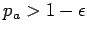for all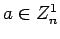.
2. There is no efficient algorithm with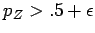It would seem that (1) is not as strong as (2). Note that (2) would rule out an algorithm with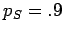and. This would be something that says this is a square'' most of the time, occasionally correctly identifying a pseudo-square. However, the paper (p. 293) shows that (1) implies (2).

Suppose we are given an algorithm. We estimatewith high probability using the techniques in Section 7.4. To take a specific example, we will assume we find,. We want to test whether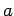is a square. Run the algorithm on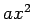for 1000 randomly chosen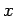. Ifis a square, the algorithm will say this is a square''times. Ifis a pseudo-square, the answer will be this is a square''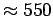times.

Translated from LaTeX by Scott Sutherland
2002-12-14